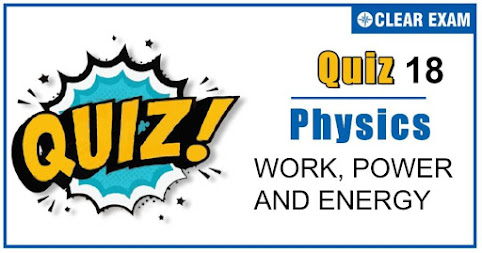## [LATEST]\$type=sticky\$show=home\$rm=0\$va=0\$count=4\$va=0

As per analysis for previous years, it has been observed that students preparing for NEET find Physics out of all the sections to be complex to handle and the majority of them are not able to comprehend the reason behind it. This problem arises especially because these aspirants appearing for the examination are more inclined to have a keen interest in Biology due to their medical background. Furthermore, sections such as Physics are dominantly based on theories, laws, numerical in comparison to a section of Biology which is more of fact-based, life sciences, and includes substantial explanations. By using the table given below, you easily and directly access to the topics and respective links of MCQs. Moreover, to make learning smooth and efficient, all the questions come with their supportive solutions to make utilization of time even more productive. Students will be covered for all their studies as the topics are available from basics to even the most advanced. .

Q1. An engine develops 10 kW of power. How much time will it take to lift a mass of 200 kgto a height of 40 m (g=10 m/sec2)?
•  4 sec
•  5 sec.
•  8 sec .
•  10 sec
Solution
P= mgh/t=10×103⇒t=(200×40×10)/(10×103 )=8 sec

Q2. A neutron having mass of 1.67×10-27 kg and moving at 108 m/s collides with a deutron at rest and sticks to it. If the mass of the deutron is 3.34×10-27 kg then the speed of the combination is?
•  2.56×103 m/s
•  2.98×105 m/s
•  3.33×107 m/s
•  5.01×109 m/s
Solution
According to law of conservation of momentum Momentum of neutron = Momentum of combination ⇒1.67×10-27×108=(1.67 ×10-27+3.34×10-27 )v ∴v=3.33×107 m/s

Q3. A ball of mass 2kg and another of mass 4kg are dropped together from a 60 feet tall building. After a tall of 30 feet each towards earth, their respective kinetic energies will be in the ratio of?
•  √2 ∶1
•  1∶4
•  1∶2
•  1:√2
Solution
Kinetic energy =1/2 mv2 As both balls are falling through same height therefore the possess same velocity But KE∝m [If v= constant] ∴(KE)1/(KE)2 =m1/m2 =2/4=1/2

Q4. 10 L of water per second is lifted from well through 20 m and delivered with a velocity of 10ms-1,then the power of the motor is?
•  1.5 Kw
•  2.5 Kw
•  3.5 Kw
•  4.5 Kw
Solution
Power ,p=(Total Energy)/t = (mgh+1/2 mv2)/t (10×10×20+1/2+10×10×10)/1 =2000+500=2500 W=2.5 KW

Q5. A bucket tied to a string is lowered at a constant acceleration of g/4. If the mass of the bucket is m and is lowered by a distance d, the work done by the string will be?
•  mgd/4
•  -3/4 mgd
•  -4/3 mgd
•  4/3 mgd
Solution
From force diagram as shown in figure mg-T=ma
T=mg-ma=mg-mg/4=3mg/4 ∴ WT= work done by tension =T .s =Ts cos⁡180°=-3mgd/4

Q6. A 20 kg ball moving with a velocity 6 ms-1 collides with a 30 kg ball initially at rest .if both of them coalesce ,then final velocity of the combined mass is ?
•  6 ms-1
•  5 ms-1
•  3.6 ms-1
•  2.4 ms-1
Solution
From conservation of momentum. Momentum before collision =Momentum after collision m1 u1+m2 u2=m1 v1+m2 v2 20×6+30×0=20v+30v ∴20×6=50v Or v=120/50=2.4ms-1

Q7. A body of mass 3 kg acted upon by a constant force is displaced by Smetre, given by relationS= 1/3 t2, where t is in second. Work done by the force in 2 seconds is?
•  8/3 J
•  19/5 J
•  5/19 J
•  3/8 J
Solution
Given that, S=1/3 t2 v=dS/dt=2/3 t; a=(d2 S)/(dt2 )=2/3 F=ma=3×2/3=2N; Work=2×1/3 t2 At t=2 Work =2×1/3×2×2=8/3 J

Q8. The blocks of mass m each are connected to a spring of spring constant k as shown in figure. The maximum displacement in the block is?
•  √((2mv2)/k)
•  √((mv2)/k)
•  2√((mv2)/k)
•  2√(k/(mv2 ))
Solution
1/2 kx2=1/2 mv2+1/2 mv2=mv2 x=√((2mv2)/k)

Q9. In an elastic collision of two particles the following is conserved?
•  Momentum of each particle
•  Speed of each particle
•  Kinetic energy of each particle
•  Total kinetic energy of both the particles
Solution
Total kinetic energy of both the particles

Q10. A rod AB of mass 10 kg and length 4 m rests on a horizontal floor with end A fixed so as to rotate it in vertical plane about perpendicular axis passing through A. If the work done on the rod is 100 J, the height to which the end B be raised vertically above the floor is?:
•  1.5 m
•  2.0 m
•  1.0 m
•  2.5 m## Want to know more

Please fill in the details below:

## Latest NEET Articles\$type=three\$c=3\$author=hide\$comment=hide\$rm=hide\$date=hide\$snippet=hide

Name

ltr
item
BEST NEET COACHING CENTER | BEST IIT JEE COACHING INSTITUTE | BEST NEET & IIT JEE COACHING: Work,Power and Energy Quiz 18
Work,Power and Energy Quiz 18